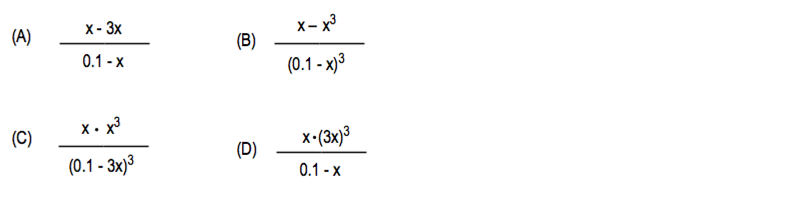# Problem: Consider this reactionAB3(g) ⇌ A(g) + 3B(g) What is the equilibrium constant expression if the intial concentration of  AB3 is 0.1 M and the equilibrium concentration of A is represented by x? Assume the intial concentrations of A and B are both zero.

83% (77 ratings)
###### Problem Details

Consider this reaction

AB3(g) ⇌ A(g) + 3B(g)

What is the equilibrium constant expression if the intial concentration of  AB3 is 0.1 M and the equilibrium concentration of A is represented by x? Assume the intial concentrations of A and B are both zero.What scientific concept do you need to know in order to solve this problem?

Our tutors have indicated that to solve this problem you will need to apply the ICE Chart concept. You can view video lessons to learn ICE Chart. Or if you need more ICE Chart practice, you can also practice ICE Chart practice problems.

What is the difficulty of this problem?

Our tutors rated the difficulty ofConsider this reactionAB3(g) ⇌ A(g) + 3B(g) What is the equi...as low difficulty.

How long does this problem take to solve?

Our expert Chemistry tutor, Jules took 1 minute and 45 seconds to solve this problem. You can follow their steps in the video explanation above.

What professor is this problem relevant for?

Based on our data, we think this problem is relevant for Professor Lekin's class at UWATERLOO.